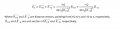#### BlackMelon

Joined Mar 19, 2015
156
Hi there!

I have a question about the Faraday's Nested Sphere Experiment, please see the attached pdf. I wonder why equation (1) and the electric field's equation ( coming after (1) ) consider only the charge Q. Why there aren't charge -Q in the equation?

Ps. I'm thinking about point charges. When you have two charges: +Q and -Q, and you want to find the electric field at point x. You need to put both +Q and -Q in an equation:BlackMelon

#### Attachments

• 60.2 KB Views: 7

#### WBahn

Joined Mar 31, 2012
29,130
Q is just a charge -- it can be positive or negative.

#### RoofSheep

Joined Mar 7, 2023
36
Hi there!

I have a question about the Faraday's Nested Sphere Experiment, please see the attached pdf. I wonder why equation (1) and the electric field's equation ( coming after (1) ) consider only the charge Q. Why there aren't charge -Q in the equation?

Ps. I'm thinking about point charges. When you have two charges: +Q and -Q, and you want to find the electric field at point x. You need to put both +Q and -Q in an equation:
View attachment 292143

BlackMelon
I think the book should have mentioned that as the inner sphere becomes smaller, the outer sphere becomes larger. The outer sphere becomes the so-called "charge at infinity". Because the distance to this charge is so large, it has no effect on the electric field generated by the point charge.

Last edited:
•BlackMelon